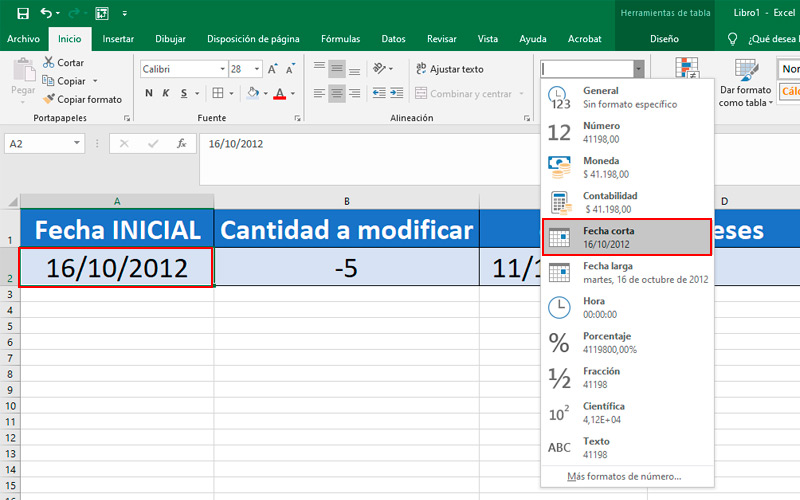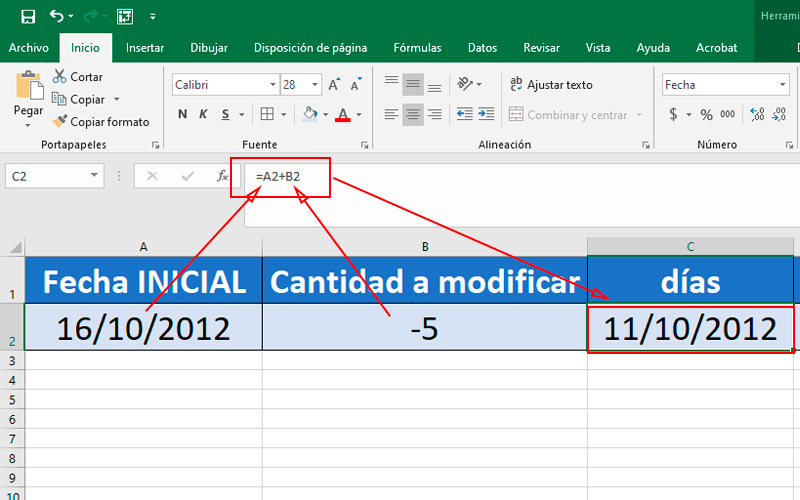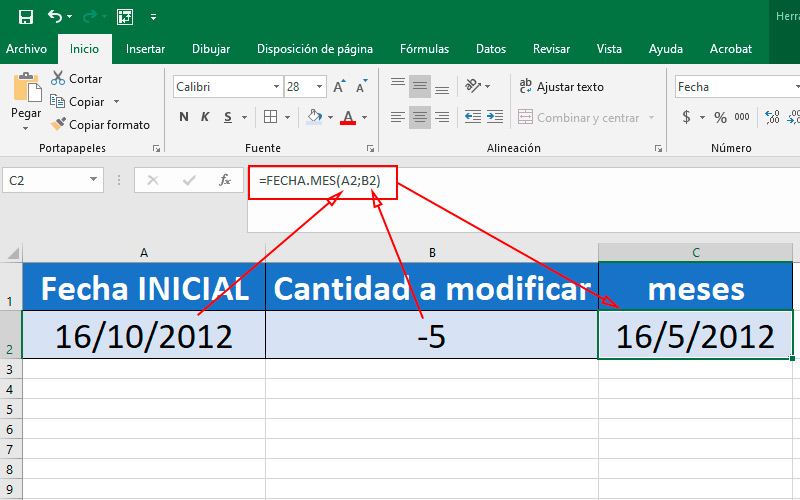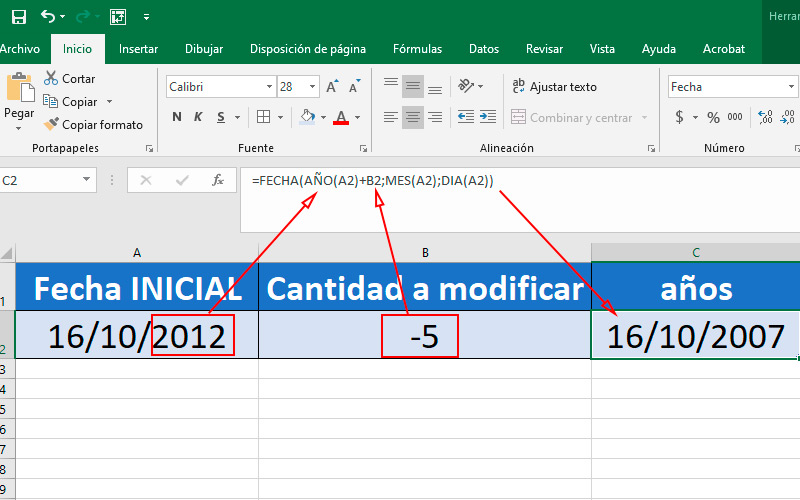Subtract Dates in Excel  Step by Step Guide ▷ 2020

Excel is software developed by Microsoft and included in the Office Suite. He himself is intended to organize data in tables, being able to perform hundreds of operations Y formulas at. In addition, it has a lots of tools and utilities that facilitates the analysis, categorization and updating of information.

One of the most used operations in this program is addition and subtraction. They are used in budgets, summaries, data analysis, etc. But on many occasions, we need compute or analyze time periods.

And in this case, perform operations of this type can be confusing. Therefore, we present the following post, where you will learn all about subtracting dates.

When is it convenient to subtract dates in an Excel document?

While add years to a given date, it is a simple task to solve, since the decimal method, like any other integer. In the case of the months and days can be a little more cumbersome. Above all, if we must add a large figure or we have to do it with various dates.

For example, if you want to postpone multiple events for three weeks, you would waste time doing the calculations manually. On the other hand, through simple functions, you can do it in a very simple way, even if it were hundreds of dates.

In addition to add weeks, it’s possible subtract them, the same happens with days or even years.

Learn step by step how to subtract dates from an Excel spreadsheet

As we mentioned before, these are very simple formulas to apply. They will serve you in all types of projects.

You just have to follow these steps:

• Open Microsoft Excel with the shortcut on the desktop or from the start menu.
• Create a new spreadsheet or access the one where you have the dates.
• In a column, may be the first, place those dates to which you want to add or subtract time.• Although, it usually be automatic, make sure all the ones you entered are in the format of “Date”, it does not matter if long or short.
• If it is not with this provision, you can replace it from the tab “Start”, in the section “Number”, change “General” by “Short date” or “Long date”.
• In the second column enter the number of days, months or years you want to modify. If you are going to subtract them add a minus sign before the number, if you are adding leave it like that. The format of this column should be kept in “General”.
• In the third column is where we will enter the function. It must have a date format.• For the days, you’ll just have to add the cell in the first column plus the cell in the second. In the example of the image it looks like this: “= A2 + B2”.
• If you need add or remove months we will use the argument DATE.MONTH which will tell the program that we want to change the referred segment.• To do this write in the cell “= DATE.MONTH (the cell that includes the date to be modified; the one that contains the figure to be added)”. In the example it is as follows “DATE: MONTH (A2; B2)”.• In case you want change the year of a single dateWe recommend doing it the traditional way. But if it were many, you can use the example function “= DATE (YEAR (A2) + B2; MONTH (A2); DAY (A2))”. In which A2 is the start date and B2 the figure to be added.

As you can see in this last action, the function separates into three segments cell A2 and add the value of B2 only the one who represents the year.

If you have any questions, leave them in the comments, we will answer you as soon as possible, and it will also be of great help to more members of the community. Thank you! 😉

Rate this post